## Solution to 1986 Problem 74

 Because no light passes through the two polarizers initially, their axes must be perpendicular to one another. Let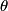$\theta$ denote the angle between the middle polarizer and the first one. Then, the middle polarizer reduces the intensity the light that passes through it by a factor of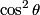$\cos^2 \theta$. And the last polarizer reduces the intensity of light that passes through it by a factor of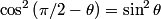$\cos^2 \left(\pi/2 - \theta \right) = \sin^2 \theta$. So, the intensity of light that passes through all three is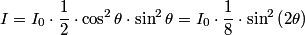\begin{align*}I = I_0 \cdot \frac{1}{2} \cdot \cos^2 \theta \cdot \sin^2 \theta = I_0 \cdot \frac{1}{8} \cdot \sin^2 \left(2 ...The factor of 1/2 comes from the fact that any polarizer reduces the intensity of unpolarized light by a factor of 1/2.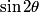$\sin 2 \theta$ is maximized when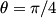$\theta = \pi/4$, and it has a maximum value of 1. So, the maximum fraction of incident power transmitted is 1/8. Hence answer (B) is correct.